# Jensen’s Inequality As An Intuition Tool

I came across a tool from mathematics called Jensen’s Inequality. I’m going to explain the rule, provide intuitive examples, then end by pointing you to real-world applications.

A warning to math whizzes — I don’t have formal math training so this post is divorced from pedagogical context. Yes, there will be numerical examples. But the real goal is for readers to recognize when the domain they are reasoning about is subject to the surprising predictions of Jensen’s Inequality. For most of us, the value of this tool is how it nudges our intuition to better predictions, not in the direct application of a formula.

Here’s where we’re going:

1. Why I found Jensen’s Inequality interesting
2. The conditions and statement of the inequality
3. An example that affects us all
4. Spotting Jensen’s in the wild

## Why I Found Jensen’s Inequality Interesting

Blindness To Exponents

Exponential phenomena confuse our brains. It has become tiresome to point out that we do not have natural intuition for growth and decay rates. Even finance folk who are apt to appreciate the idea of compounding  seem to not recognize it when the investing skin is pulled off it.

Covid is a timely example. A virus’ R0 (“R naught”) indicates how transmissable it is. Remember “Covid is the flu”. Say the flu has an R0 of 2. So for each person that contracts the flu, they infect 2 more people. Now let’s suppose Covid has an R0 of 3. Here’s how the 2 viruses would spread 1.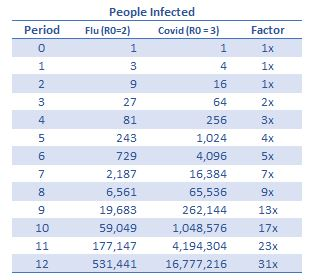R0 is a more complicated function than I’m stylizing here (it should be obvious that behavior, like masks, change it. And if a virus was super effective at replicating itself, well it would find new hosts harder to come by). My point is that even smart people will not hear the “This Is Not A Linear Phenomena” song unless their station is tuned to it. The failure to recognize non-linear domains is serious, because it leads to wildy wrong predictions. And life is prediction. We implicitly predict that the sun will rise tomorrow.

Jensen’s Inequality guides our predictions by forcing us to deliberately consider how the average input maps to the average output. When the function that maps the input to the output is non-linear, Jensen’s Inequality tells us in which direction our predictions will be biased. Stated another way: Jensen’s Inequality informs us when an average occurance is a poor predictor of the average result.

Before we get to any equations, let’s predict the outcome of a simple game.

### Dice Payoff

Imagine a game, you stake \$1, then roll 2 dice. Whatever the roll returns times your stake amount is how much money you make. That’s the payoff function.

So if you roll a five you receive \$5.

Question 1: On average, how much do you expect to get paid?

This is a straightforward expected value problem. You get paid the weighted average of all the outcomes or on average \$7.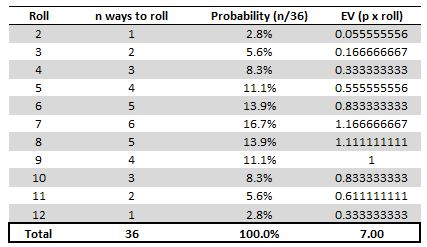The average value that you roll will correspond to the average value of the payoff function. If that sounds obvious, that’s the point. So far, so good.

Question 2: If you staked \$100, what would you predict the average payoff from playing the game?

A quick way to estimate that would be to ask yourself, “what do we expect to roll on average?” then multiply that by the staked amount. In this case, we roll a 7 on average, and since the staked amount is \$100 then on average when we play this game we expect to be paid out \$700.

That prediction is correct. We can brute force the expected value of the payoffs.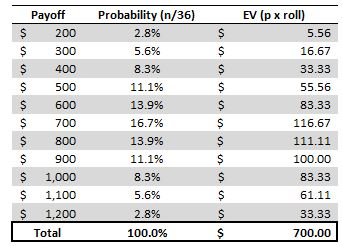At this point, things are feeling pretty obvious and redundant, but let me remind you what we did to just answer Question 2. We used a shortcut. We took the expected value of the roll, which was an input, to estimate the expected value of the payoff function or the output. The shortcut worked because the payoff function was linear. We are just scaling the expected input by the staked amount since the function is simply (dice roll x staked amount). This kind of payoff function exists all around us. When you buy a stock, your p/l function is just change in stock price x share quantity. The “staked amount” in our example performs the same scaling role as share quantity.

You can feel the twist coming.

Question 3: Same game but we change the payoff function to (staked amount) x (dice roll)2. What’s the average payoff?

First, what does our shortcut predict? Let’s say we bet \$1 again. Since the average value we roll is a 7, then we expect the average payoff to be \$72 x \$1. So we expect the average payoff of this game to be \$49.

As you may have guessed from the unsubtle narrative arc, \$49 is the wrong answer. Our shortcut doesn’t work. Brute force method: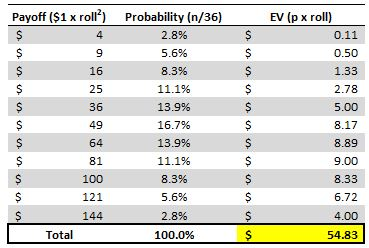It turns out the expected value or average result from the squared game is \$54.83, a higher value than what we would predict if we took the average value of the input and simply applied the squared function to it.

It’s intuitive to take the average value of an input, apply a function to it and call that the “expected value of the function”. It turns out that if the function we run the input through is exponential, our estimate will be wrong. So in service of becoming better at making estimates on the fly, we should get better at thinking about what kind of function we are running an input through and if our prediction is likely to be biased higher or lower than the actual expected value of the payoff function.

With that long intro we can now turn to Jensen’s Inequality and its practical applications.

## A Look At Jensen’s Inequality

### …if f(x) is convex

Let’s try saying this in words several ways, assuming f(x) is convex (a term I will address in a bit):

• The expected value of a function is greater than or equal to the function applied to the expected value of the input.

• The average value of a function is greater than or equal to the function applied to the average input.

• Returning to dice…the [weighted] average of all the squares is greater than the square of the average roll.

In practice this means, you cannot estimate the average value of the function based on the average value of the input IF the function is exponential.

### Convex

Let’s address the term “convex”. You know what it is visually.Mathematically, a convex function has a second derivative that is greater than 0, meaning as X increases the slope itself increases. The steepness of the chart is increasing.

If we go back to the dice example and consider the convex payoff function, we can see the average value of the payoff function of \$54.76 is greater than the payoff (\$49) at the average roll. In other words, the convex function ensured that:

average value of all payoffs > the payoff of the average roll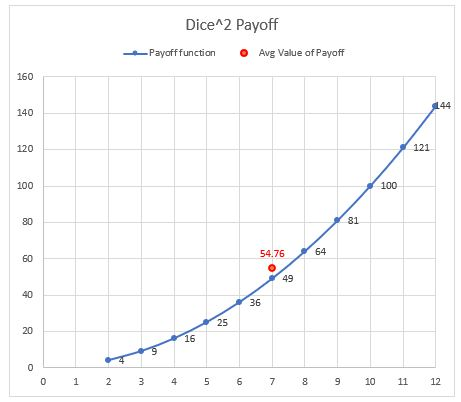### Concave

For concave functions, like y = sqrt(x), we have a positive slope, but the slope is decreasing as x increases. The second derivative is negative. Let’s look at a concave case for the dice game by making the payoff function = sqrt(roll).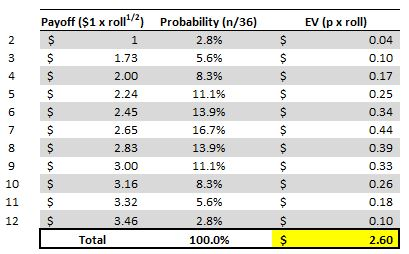Notice that the average value of the payoff, if you stake \$1, is \$2.60. But if you tried to predict the expected payoff by using the shortcut of taking the square root of the average roll you’d predict \$2.65 which is the sqrt(7).

Wait a minute. The prediction this time overshot the true expected value of the function?!

That’s correct. If you multiply one side of an inequality by -1 you flip the sign…a convex function can be flipped to concave by flipping the sign as well. So a concave function flips the sign of Jensen’s Inequality, making the overshoot the expected result.

Visualizing the concave payoff:Let’s practice with a highly stylized example I made up, but relates to something we all intuitively feel.

## An Intuitive Example That Affects Us All: Traffic!

We are celebrating a big W, so it’s time to take the kids to Sizzler. We’re going to drive. Sizzler is 10 minutes away + some extra time depending on how many cars are on the road. Let’s keep things very simple and assume the number of cars that can be on the road is 10, 20, 30, 40, or 50 and with equal probability. None of these quantities is enough to slow the flow of traffic to a halt, but the impact of the extra cars is not linear.

We’ll create a function called “time to destination” denominated in seconds and make it a function of “cars on the road”:

f(cars on the road) = x2 + 600

Let’s play “How long will it take to get to Sizzler?”

Before you discovered this post, you likely would have said 25 minutes. Why? Since we can have 10, 20, 30, 40, or 50 cars all with equal probability, then on average we expect to see 30 cars on the road.

302 + 600 = 1500 seconds or 25 minutes.

But because we know about Jensens’s Inequality we:

1. recognize the traffic output function is convex
2. realize that the expected value of the traffic function will be greater than sticking the average number of cars in the road into the traffic function

Enlightened, we instead estimate that on average it will take longer than 25 minutes to pounce on that glorious salad bar with the popcorn shrimp.

How much longer? Brute force tells us 28.3 minutes!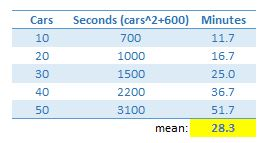## Spotting Jensen’s Inequality In The Wild

Here’s a few common applications that abide Jensen’s Inequality

• Geometric mean ≤ arithmetic mean

I’ll need to point you to an actual math person. See his beautiful derivation on YouTube. It’s easy to follow along and quite clever. The key to this inequality is recognizing that the geometric mean, which takes the nth root, is concave just like the sqrt(x) function.

It’s worth noting that the LN(x) function is also concave so when you are in price space we know that the LN(average price) > the average of the LN(prices). Same idea as the geometric means, concavity flips the sign of Jensens.

• Call options

Here’s a generic chart3.A call option is convex payoff function with respect to the stock price. Its first derivative with respect to the price of a stock is delta which is always positive. In other words, as the stock price goes up, all else equal, the call option always goes up (the slope or delta of a way OTM option is 0 so it’s possible for the call to not change in value, but that’s the lower bound). The second derivative with respect to stock price is gamma and it also is always at least worth 0. That means that as a stock price increases the delta or slope itself increases (or hits the zero lower bound).

In options land, stock prices are assumed to be lognormally distributed. This is a reasonable distribution since a stock is bounded by zero and stretches to infinity. The expected value of a stock is the current stock price (in a no arbitrage framework)4.

Now let’s go back to Jensen’s Inequality:

### …if f(x) is convex

Substituting words:

The expected or average value of a call for all possible prices of the stock (of course weighted by their probabilities) will be greater than the value of the call based on the stock being at it’s expected price (which is just the current price in Black Scholes).

In other words, the average value of a call will be higher than the value of a call in the average scenario.

It is easier to see with a binary stock (as opposed to a lognormally distributed stock). Suppose a binary stock is \$10. That’s its expected value. Suppose now that the expected value is driven by the fact that it’s 90% to be worth 0 and 10% to be worth \$100. The 50 strike call is worthless in the average scenario (since \$10 is the weighted avg of the scenarios…again that’s what a stock price is by definition).

But the weighted average of its value over both scenarios is \$5 (90% x 0 + 10% x (100-50))

Again, the average value of a call will be higher than the value of a call in the average scenario.

So next time somebody uses the logic that they don’t buy options because most options expire worthless you can remind them that the typical outcome is not what drives the value of options. Instead, you should care about the average value of the option over all scenarios.

By the way, nothing I said here is revelatory. It’s not like any serious person thinks OTM options are worthless in the first place and just prices options based on the stock’s expected value.

• Technology

Here’s a qualitative one. Suppose teacher skill follows a bell curve. Skill is the independent variable. Our X. Our payoff function is going to be how many people the teacher can effectively educate. A great teacher will impact a higher percentage of the students they actually come into contact with because they are more effective. If we had to predict the payoff, we might be tempted to apply a function to the average teacher. This would be like taking the 7 we rolled and running it through some payoff function.

But if we consider the average value of the teacher payoff function for all level of teachers we will find that prior estimate to be wildy too low. Sal Khan comes to mind. He literally broke the function despite being just a good or even great teacher but very much on the bell curve.

The point is that technology leads to a huge range of possible payoff functions semingly extending to billions. Estimates of output based on the average input will fail to appreciate the convexity of some of the payoff functions in a hyper-connected world network.

This might not map perfectly to Jensen’s Inequality but it was a thought I had as I turned these concepts over in my mind.

• Investing

In Convexity in DCFs, Robert Martin uses this intuition to show why choosing an asset that increases cash flow by 30% on average can be worth more than an asset which always grows at 30%. The average of the growth rate is a poor guide to the average output of the function (the function being the total return) because the function is convex with respect to growth rate.

The math of compounding is non-linear so the impact of a 40% growth rate is greater than 4/3 of a 30% growth rate. Compounding is not intuitive, but if we keep Jensen’s inequality in mind, we can quickly realize that our minds will misdiagnose the impact of the input parameters (in this case 30% vs 40%) on final payoff functions because compounding is a convex phenomena. By remembering that Jensen’s inequality exists, we remember to slow down before estimating the end result of compounded inital inputs even if the inputs don’t seem to differ by large amounts.

## Takeways

Be careful when trying to make estimates of how a function or process will payoff based upon the average input. If the function has exponential dynamics, Jensen’s Inequality tells us that the weighted average value of the function will not coincide with average input you feed it.

If the function is convex, the average input will underestimate the average output. If the function is concave, it will overestimate the average output.

1. assuming nobody ever healed
2. I learned about Jensen’s Inequality just last week from this YouTube clip. The author used the same example of dice, but if you browse my writing you’ll find I use the same example all the time. The point of these posts is to ELI5 and dice are the universal demo.
3. I borrowed this from here.
4. If we assume no carry costs or dividends

## 2 thoughts on “Jensen’s Inequality As An Intuition Tool”

1.chris stucchio says:

Minor nitpick – a convex function doesn’t need to be differentiable at all. The actual definition is that if 0

A simple example of a non-differentiable function that satisfies this is the actual payoff of a call option you have graphed, i.e. f(x) = 0, x strike.

1.editor says:

Thank you Chris…on my way to looking up the definition of a convex function (I think you tried to write it but it looks cut off)